• 合并排序（归并排序）
千次阅读
2021-05-13 09:28:36

合并排序，大致思想便是先将数组中的元素拆分成若干小部分，然后再将这些小部分按照顺序进行重新组合，从而实现排序。很明显，这里用到了分治法的思想，即将一个大问题分成若干个相同的小问题，因为问题规模变小了，所以解决问题的难度也随之减小，试想一下，对一个拥有10000个元素的数组进行排序简单还是对一个只拥有1个元素的数组进行排序简单？答案很明显。

对于合并排序，将大问题分成若干小问题是一件很容易的事情，只需要不断递归即可，其中最主要的难点就是将这些小问题解决之后，如何再将这些小问题合并起来，若是处理不好，很可能就导致算法失败。那么接下来就开始正式的讲解。

## 先上伪代码

因为伪代码没有其他语言的语法限制，能够有助于我们更好地将注意力放在算法上，所以这里我们先用伪代码来讲述一下主要思想：

algorithm mergeSort(A[0..n-1])
//递归调用mergeSort来对数组A[0..n-1]排序
//输入：一个可排序的数组A[0..n-1]
//输出：非降序排列的数组A[0..n-1]
if n > 1  //将数组中的n个元素分成n组
copy A[0..(n/2)] to B[0..(n/2)]
copy A[(n/2)..n-1] to C[0..(n/2)-1]
mergeSort(B[0..(n/2)])
mergeSort(C[0..(n/2)-1])
merge(B, C, A)  //将B和C合并到A中

algorithm merge(B[0..n-1], C[0..m-1], A[0..n+m-1])
//将两个有序数组B和C合并到A中
//输入：两个有序数组和一个待整合数据的数组
//输出：A[0..n+m-1]中已经有序存好了B和C中的数据
i <- 0;j <- 0;k <- 0
while i < n and j < m do
if B[i] <= C[j]
A[k] <- B[i]
i <- i + 1
else
A[k] <- C[j]
j <- j + 1
k <- k + 1
if i = n  //说明B中的所有元素已经存放到A中，即C中的部分元素为存入到A中
copy C[j..m-1] to A[k..n+m-1]
else
copy B[i..n-1] to A[k..n+m-1]


因为不管怎样，一个元素的数组绝对是最好排序的，因为不需要排序，所以我们一开始就需要将整个数组分成n个组，之后再慢慢地合并，如下图便是合并排序的一个例子：## 代码实现

这里我是用的C++来实现该算法：

关键部分：

void merge(int* B, int lenB, int* C, int lenC, int* A, int lenA) {
int i = 0, j = 0, k = 0;
while (i < lenB && j < lenC) {
if (B[i] <= C[j]) A[k++] = B[i++];
else A[k++] = C[j++];
}
if (i == lenB) {
while (j < lenC) A[k++] = C[j++];
} else {
while (i < lenB)A[k++] = B[i++];
}
}

void mergeSort(int* A, int lenA) {
if (lenA > 1) {
int n1 = lenA / 2;
int n2 = lenA - n1;
int* B = (int*)malloc(sizeof(int) * n1);
int* C = (int*)malloc(sizeof(int) * n2);
for (int i = 0; i < n1; i++) {
B[i] = A[i];
}
for (int i = 0; i < n2; i++) {
C[i] = A[n1 + i];
}
mergeSort(B, n1);
mergeSort(C, n2);
merge(B, n1, C, n2, A, lenA);
free(B);
free(C);
}
}


然后在main()函数中调用：

int main() {
cout << "排序前的数组:" << endl;
for (int i = 0; i < 10; i++) {
cout << A[i] << " ";
}cout << endl;

mergeSort(A, 10);

cout << "排序后的数组:" << endl;
for (int i = 0; i < 10; i++) {
cout << A[i] << " ";
}cout << endl;
return 0;
}


运行结果如下：## 复杂度分析

合并排序的复杂度要分成两部分来看，一部分是分治，另一部分则是合并，

其中合并部分，最差执行n-1次比较，最优执行n/2次比较，都是O(n)，

因此得到如下公式：
再由主定理可得合并排序的时间复杂度为O(nlogn)，并且无论是最优最差还是平均，复杂度都是O(nlogn)。

接下来我们再来看看合并排序的空间复杂度，

我们同样可以得到如下公式：
所以我们也可以马上知道合并排序的空间复杂度也为O(nlogn)。

由此我们也可以发现，虽然合并排序的时间复杂度比较好，但是空间复杂度确实太高了，相比冒泡排序、选择排序这些在位排序而言，占用的额外空间实在是太多了。

因此针对合并排序的改进方向便是减小空间复杂度。

## 参考资料

《算法设计与分析基础》第三版以及老师的课件算法
更多相关内容
• 现在就用递归算法，采用上面的分治思想来解合并排序。  合并排序（非降序） 分解：把合并排序分解成与两个子问题 伪代码： 代码如下:MERGE_SORT(A, begin, end) if begin < end  then mid<- int((begin + ...
• 　现在用递归算法，采用上面的分治思想来解合并排序。 　合并排序（非降序） 　分解：把合并排序分解成与两个子问题 　伪代码：  MERGE_SORT(A， begin， end)  if begin < end  then mid<- int...
• 归并排序也称合并排序，其算法思想是将待排序序列分为两部分，依次对分得的两个部分再次使用归并排序，之后再对其进行合并。仅从算法思想上了解归并排序会觉得很抽象，接下来就以对序列A, A[l]…, A[n-1]进行升序...
• 本源代码为C语言编写的合并排序算法实现，代码内数组初始为1-9，如有需要变动的请注意merge函数中的temp[]数组的大小必须和你设置的数组大小相同。
• 使用插入排序算法对输入的n个整数，按照从小到大的顺序排序。 Input Description 第一行输入一个整数n（0）。 第二行输入n个整数。 Output Description 输出排序后的整数，每个整数之间以一个空格分隔。注意：最后...
• 实现并验证合并排序算法； Ex2：实现并验证快速排序算法 Ex3：用递归与分治的方法设计并实现寻找第k小元素算法
• /************合并排序算法的实现******************/ int main() { int p,q,r; printf("合并排序算法的实现:\n"); printf("请输入p、q、r的值(输入格式1,12,13):"); scanf("%d,%d,%d",&p,&q,&r); printf("p=%...
• 文件的输入可以来自 excel、.mat 或 .txt 文件。 如果发现要排序的数组是多维数组，则必须提供更多信息。 还可以选择一次对行或列进行选择性或集体排序matlab
• 主要介绍了C++实现合并排序的方法,实例分析了合并排序的原理与相关实现技巧,需要的朋友可以参考下
•C++
• matlab开发-多维数组的合并排序。使用合并排序技术对单个或多维数组进行排序。
• 实现并验证合并排序算法； 实现并验证快速排序算法（参考习题5.2第7a题，P140）； 用递归与分治的方法设计并实现寻找第k小元素算法
• 合并排序算法C语言源程序，合并排序算法就是将多个有序数据表合并成一个有序数据表，进行两两合并和数据大小比较，算法程序亲测可用。
• 里面有详细的插入排序，快速排序，合并排序和选择排序的代码。 排序算法测试实验通过设计测试数据集，编写测试程序，用于测试三种算法的正确性，三种算法在不同复杂性上的表现（最好情况、最差情况、平均情况），三...
• 针对200000长度的数组，采用插入排序和合并排序，对比两种算法的时间复杂度
• c语言合并排序算法 合并排序算法 (Merge Sort Algorithm) Merge Sort follows the rule of Divide and Conquer to sort a given set of numbers/elements, recursively, hence consuming less time. 合并排序遵循...

c语言合并排序算法

Merge Sort follows the rule of Divide and Conquer to sort a given set of numbers/elements, recursively, hence consuming less time.

合并排序遵循分而治之的规则，以递归方式对一组给定的数字/元素进行排序，从而减少了时间。

In the last two tutorials, we learned about Selection Sort and Insertion Sort, both of which have a worst-case running time of O(n2). As the size of input grows, insertion and selection sort can take a long time to run.

在最后的两个教程中，我们了解了选择排序和插入排序，这两个类的最坏运行时间均为O(n 2 ) 。 随着输入大小的增长，插入和选择排序可能需要很长时间才能运行。

Merge sort , on the other hand, runs in O(n*log n) time in all the cases.

另一方面，合并排序在所有情况下均以O(n*log n)时间运行。

Before jumping on to, how merge sort works and it's implementation, first lets understand what is the rule of Divide and Conquer?

在继续之前，合并排序如何工作及其实现，首先让我们了解分而治之的规则是什么？

## 分而治之 (Divide and Conquer)

If we can break a single big problem into smaller sub-problems, solve the smaller sub-problems and combine their solutions to find the solution for the original big problem, it becomes easier to solve the whole problem.

如果我们可以将一个大问题分解为较小的子问题，解决较小的子问题，然后将它们的解决方案结合起来以找到原始大问题的解决方案，那么解决整个问题就变得更加容易。

Let's take an example, Divide and Rule.

让我们以Divide and Rule为例。

When Britishers came to India, they saw a country with different religions living in harmony, hard working but naive citizens, unity in diversity, and found it difficult to establish their empire. So, they adopted the policy of Divide and Rule. Where the population of India was collectively a one big problem for them, they divided the problem into smaller problems, by instigating rivalries between local kings, making them stand against each other, and this worked very well for them.

当英国人来到印度时，他们看到一个宗教信仰不同的国家和睦相处，工作勤奋但天真的公民，多元而团结，发现很难建立自己的帝国。 因此，他们采取了分而治之的政策。 在印度人口集体对他们来说是一个大问题的地方，他们通过煽动地方国王之间的对抗，使他们相互对抗，将问题分为较小的问题，这对他们来说非常有效。

Well that was history, and a socio-political policy (Divide and Rule), but the idea here is, if we can somehow divide a problem into smaller sub-problems, it becomes easier to eventually solve the whole problem.

那是历史，是一项社会政治政策(“ 分而治之” )，但是这里的想法是，如果我们能够以某种方式将一个问题分解为较小的子问题，则最终解决整个问题变得更加容易。

In Merge Sort, the given unsorted array with n elements, is divided into n subarrays, each having one element, because a single element is always sorted in itself. Then, it repeatedly merges these subarrays, to produce new sorted subarrays, and in the end, one complete sorted array is produced.

Merge Sort中 ，给定的具有n元素的未排序数组被划分为n个子数组，每个子数组都有一个元素，因为单个元素始终在自身中进行排序。 然后，它反复合并这些子数组，以生成新的排序后的子数组，最后生成一个完整的排序后的数组。

The concept of Divide and Conquer involves three steps:

分而治之的概念涉及三个步骤：

1. Divide the problem into multiple small problems.

鸿沟的问题分成多个小的问题。

2. Conquer the subproblems by solving them. The idea is to break down the problem into atomic subproblems, where they are actually solved.

通过解决他们征服的子问题。 想法是将问题分解为原子子问题，并在其中实际解决。

3. Combine the solutions of the subproblems to find the solution of the actual problem.

结合子问题的解决方案以找到实际问题的解决方案。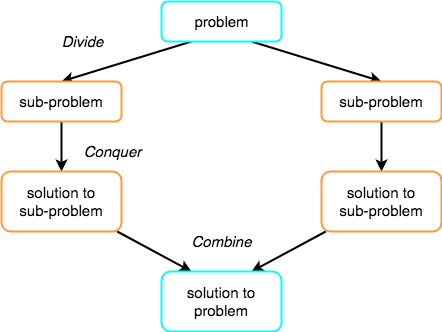## 合并排序如何工作？ (How Merge Sort Works?)

As we have already discussed that merge sort utilizes divide-and-conquer rule to break the problem into sub-problems, the problem in this case being, sorting a given array.

正如我们已经讨论的那样，合并排序利用分而治之的规则将问题分解为子问题，在这种情况下，问题是对给定数组进行排序

In merge sort, we break the given array midway, for example if the original array had 6 elements, then merge sort will break it down into two subarrays with 3 elements each.

在归并排序中，我们将给定的数组中途中断，例如，如果原始数组有6元素，则归并排序会将其分解为两个每个具有3元素的子数组。

But breaking the orignal array into 2 smaller subarrays is not helping us in sorting the array.

但是将原始数组分成2个较小的子数组并不能帮助我们对数组进行排序。

So we will break these subarrays into even smaller subarrays, until we have multiple subarrays with single element in them. Now, the idea here is that an array with a single element is already sorted, so once we break the original array into subarrays which has only a single element, we have successfully broken down our problem into base problems.

因此，我们将把这些子数组分解为更小的子数组 ，直到我们拥有多个包含单个元素的数组 。 现在，这里的想法是已经对具有单个元素的数组进行了排序，因此一旦将原始数组分解为仅具有单个元素的子数组，我们就可以成功地将问题分解为基本问题。

And then we have to merge all these sorted subarrays, step by step to form one single sorted array.

然后，我们必须逐步合并所有这些排序后的子数组，以形成一个单独的排序后的数组。

Let's consider an array with values {14, 7, 3, 12, 9, 11, 6, 12}

让我们考虑一个值{14, 7, 3, 12, 9, 11, 6, 12} 14，7，3，12，9，9，11，6，12 {14, 7, 3, 12, 9, 11, 6, 12}的数组

Below, we have a pictorial representation of how merge sort will sort the given array.

下面，我们以图形方式表示合并排序将如何对给定数组进行排序。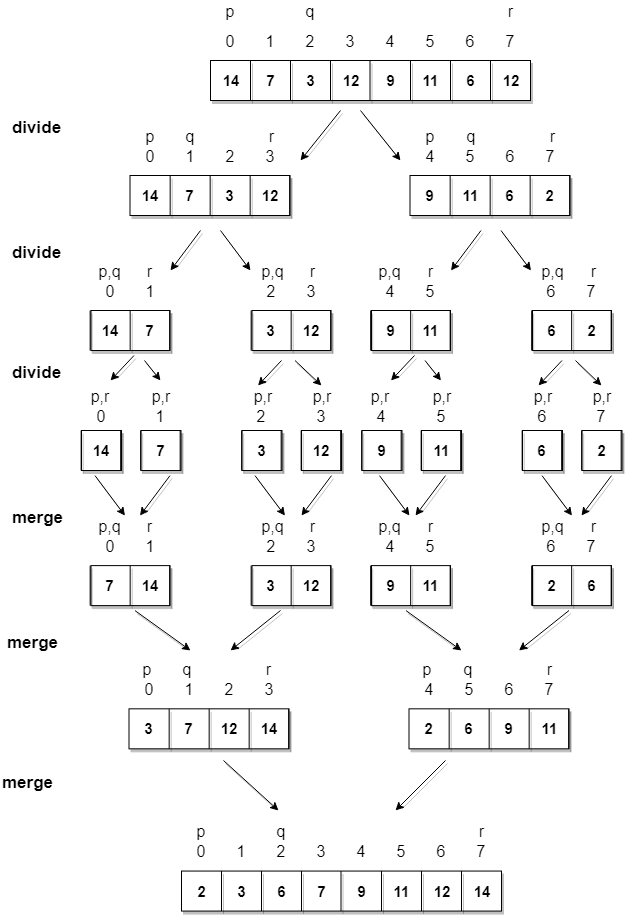In merge sort we follow the following steps:

在合并排序中，我们遵循以下步骤：

1. We take a variable p and store the starting index of our array in this. And we take another variable r and store the last index of array in it.

我们采用变量p并在其中存储数组的起始索引。 然后，我们使用另一个变量r并将数组的最后一个索引存储在其中。

2. Then we find the middle of the array using the formula (p + r)/2 and mark the middle index as q, and break the array into two subarrays, from p to q and from q + 1 to r index.

然后，我们使用公式(p + r)/2找到数组的中间，并将中间索引标记为q ，然后将该数组分为两个子数组，从pq和从q + 1r索引。

3. Then we divide these 2 subarrays again, just like we divided our main array and this continues.

然后，我们再次划分这2个子数组，就像划分主数组一样，这种情况将继续。

4. Once we have divided the main array into subarrays with single elements, then we start merging the subarrays.

一旦将主数组划分为具有单个元素的子数组，我们便开始合并子数组。

## 实现合并排序算法 (Implementing Merge Sort Algorithm)

Below we have a C program implementing merge sort algorithm.

下面我们有一个实现合并排序算法的C程序。

/*
a[] is the array, p is starting index, that is 0,
and r is the last index of array.
*/

#include <stdio.h>

// lets take a = {32, 45, 67, 2, 7} as the array to be sorted.

// merge sort function
void mergeSort(int a[], int p, int r)
{
int q;
if(p < r)
{
q = (p + r) / 2;
mergeSort(a, p, q);
mergeSort(a, q+1, r);
merge(a, p, q, r);
}
}

// function to merge the subarrays
void merge(int a[], int p, int q, int r)
{
int b;   //same size of a[]
int i, j, k;
k = 0;
i = p;
j = q + 1;
while(i <= q && j <= r)
{
if(a[i] < a[j])
{
b[k++] = a[i++];    // same as b[k]=a[i]; k++; i++;
}
else
{
b[k++] = a[j++];
}
}

while(i <= q)
{
b[k++] = a[i++];
}

while(j <= r)
{
b[k++] = a[j++];
}

for(i=r; i >= p; i--)
{
a[i] = b[--k];  // copying back the sorted list to a[]
}
}

// function to print the array
void printArray(int a[], int size)
{
int i;
for (i=0; i < size; i++)
{
printf("%d ", a[i]);
}
printf("\n");
}

int main()
{
int arr[] = {32, 45, 67, 2, 7};
int len = sizeof(arr)/sizeof(arr);

printf("Given array: \n");
printArray(arr, len);

// calling merge sort
mergeSort(arr, 0, len - 1);

printf("\nSorted array: \n");
printArray(arr, len);
return 0;
}

Given array: 32 45 67 2 7 Sorted array: 2 7 32 45 67

给定数组：32 45 67 2 7排序数组：2 7 32 45 67

### 合并排序的复杂度分析 (Complexity Analysis of Merge Sort)

Merge Sort is quite fast, and has a time complexity of O(n*log n). It is also a stable sort, which means the "equal" elements are ordered in the same order in the sorted list.

合并排序非常快，并且时间复杂度为O(n*log n) 。 这也是一种稳定的排序方式，这意味着“相等”元素在排序列表中的排序顺序相同。

In this section we will understand why the running time for merge sort is O(n*log n).

在本节中，我们将理解为什么合并排序的运行时间为O(n*log n)

As we have already learned in Binary Search that whenever we divide a number into half in every stpe, it can be represented using a logarithmic function, which is log n and the number of steps can be represented by log n + 1(at most)

正如我们在二元搜索中所学到的，每当我们在每个stpe中将数字分为一半时，就可以使用对数函数来表示，即log n ，步数可以由log n + 1表示(最多)

Also, we perform a single step operation to find out the middle of any subarray, i.e. O(1).

同样，我们执行一步操作来找出任何子数组的中间，即O(1)

And to merge the subarrays, made by dividing the original array of n elements, a running time of O(n) will be required.

为了合并通过划分n元素的原始数组而形成的子数组，将需要O(n)的运行时间。

Hence the total time for mergeSort function will become n(log n + 1), which gives us a time complexity of O(n*log n).

因此， mergeSort函数的总时间将变为n(log n + 1) ，这使我们的时间复杂度为O(n*log n)

Worst Case Time Complexity [ Big-O ]: O(n*log n)

最坏情况下的时间复杂度[Big-O]： O(n * log n)

Best Case Time Complexity [Big-omega]: O(n*log n)

最佳情况下的时间复杂度[Big-Omega]： O(n * log n)

Average Time Complexity [Big-theta]: O(n*log n)

平均时间复杂度[Big-theta]： O(n * log n)

Space Complexity: O(n)

空间复杂度： O(n)

• Time complexity of Merge Sort is O(n*Log n) in all the 3 cases (worst, average and best) as merge sort always divides the array in two halves and takes linear time to merge two halves.

在所有3种情况下(最差，平均和最佳)，合并排序的时间复杂度均为O(n*Log n) ，因为合并排序始终数组分为两半，并花费线性时间来合并两半。

• It requires equal amount of additional space as the unsorted array. Hence its not at all recommended for searching large unsorted arrays.

它需要与未排序数组相等数量的额外空间 。 因此，完全不建议搜索大型未排序的数组。

• It is the best Sorting technique used for sorting Linked Lists.

这是用于对链表进行排序的最佳排序技术。

c语言合并排序算法

展开全文算法 java 数据结构 python 机器学习
• 自然合并排序算法，对合并排序算法进行进一步的优化java
• 合并排序算法排序过程 每个程序员都需要了解他们的算法和数据结构。 在研究它们时，您需要确保确切了解它的功能，时间和空间的复杂性以及采用这种方式的原因，并且不仅能够对其进行编码，而且能够手动执行。 这就是...

合并排序算法排序过程

每个程序员都需要了解他们的算法和数据结构。 在研究它们时，您需要确保确切了解它的功能，时间和空间的复杂性以及采用这种方式的原因，并且不仅能够对其进行编码，而且能够手动执行。 这就是基本算法的全部意义。

欢迎来到基本算法的第一次安装，今天在这里，我们将介绍合并排序。 合并排序是用于对大型数据集进行排序的最可靠的一致且性能最佳的排序算法之一，比起快速排序平均而言， 最糟糕情况下执行的比较少了近40％。 因此，让我们深入研究一下此算法是什么。

## 合并排序算法

首先，让我们看一个简单的数字数组，这些数字是乱序的，我们要对其进行排序：

var arr = [ 27 , 369 , 63 , 126 , 252 , 990 , 54 , 18 ]

合并排序算法可以采用这些数字，并以一致且有效的方式将它们按大小从小到大或从小到大的顺序排列。 合并排序采用递归的分治方法。 也就是说，它通过调用自身将数组分解为越来越小的数组，直到每个数组中只有一个元素为止。 然后，它一次比较两个数字，将它们排序，然后将它们返回给自己，因为它从递归调用函数中退出。 因此，我们上面的数组变成了以下子数组：

[[27 , 369 , 63 , 126 ],
[ 252 , 990 , 54 , 18 ]]

并再次放入：

[[[27 , 369 ], [ 63 , 126 ]], [[ 252 , 990 ], [ 54 , 18 ]]]

最后一次：

[[[[27 ], [ 369 ]], [[ 63 ], [ 126 ]]], [[[ 252 ], [ 990 ]], [[ 54 ], [ 18 ]]]]

将元素分类到子数组中后，需要对每对元素进行求值，以便在合并在一起时，左值小于右值。 在此示例中，仅最后一对单元素数组在左侧具有较大的值，因此, 将合并为[18,54]

[[[27 , 369 ], [ 63 , 126 ]], [[ 252 , 990 ], [ 18 , 54 ]]]

在最小的元素保持在最多嵌套子数组的index 的情况下，现在可以有效地按顺序合并子数组。 为此，比较两个数组的第一个元素。 然后，从子数组中取出较小的元素，然后将它们放入要返回的数组中，同时将它们从要比较的数组中取出。

因此，比较数组[27, 369][63, 126] ，我们只需要查看数组的第一项即可。 由于27 <63，因此27将被从第一个数组中取出，并将其添加到我们将返回的数组中。 这使我们可以比较, [63,126] 。 我们只需要再次查看第一个元素，然后看下一个63，因此我们返回的数组为[27,63] ，我们还有, 进行比较。 然后，我们添加126，只剩下369，因此我们将其添加。 总而言之，步骤如下所示：

[27 , 369 ] [ 63 , 126 ]
[]

[ 369 ] [ 63 , 126 ]
[ 27 ]

[ 369 ] [ 126 ]
[ 27 , 63 ]

[ 369 ]
[ 27 , 63 , 126 ]

[ 27 , 63 , 126 , 369 ]

第二次迭代后，数组如下所示：

[[27 , 63 , 126 , 369 ], [ 18 , 54 , 252 , 990 ]]

然后以完全相同的方式再次合并数组，将27与18进行比较，然后将27与54进行比较，再将63与54进行比较，依此类推，直到对整个数组进行排序并返回为止。

当我前面提到需要对每个对进行评估，并且第一步只需要交换, ，这两个对都保存在自己的数组中。 这意味着可以创建一个函数来比较所有实例中的数字，方法是比较两个数组的索引0处的元素，然后在将较小的项添加到返回数组中时将其删除。 这将一直运行到其中一个数组为空，而另一个数组的其余元素将附加到返回元素的末尾。

数组将以这种方式递归合并在一起，直到只剩下一个数组，然后将其完全排序。 在某种程度上，您可以将其分为两个基本步骤：

1. 合并两个数组：合并两个数组。 比较其中两个的第一个元素。 应该从该数组中删除较小的元素，并将其添加到我们要返回的数组末尾。 当从一个数组中删除所有元素时，只需将其他元素的其余部分添加到已排序的数组中并返回它。
2. 分而治之：取一个数组。 找到它的中间。 将其左侧的所有内容传递到divide and conquer函数中，并将其右侧的所有内容传递给divide and conquer函数（因此该函数已被同时馈入了一半），直到只有一个。 然后，开始合并两个数组。

## 时间复杂度和效率

类似于堆排序，合并排序在最佳和最差情况下的时间复杂度为O（n * log（n）） 。 之所以不会改变，是因为它会在调用列表时执行对列表进行完全排序所需的所有操作。 合并排序获取列表时，对列表进行排序并不重要。 它可以已经排序，也可以尽可能不排序，但是合并排序仍然必须执行列表的O（log（n））划分和O（n）比较。

这与诸如冒泡排序（Bubble Sort）之类的其他算法形成对比，该算法以递归方式遍历列表直到对其进行排序。 如果给出理想的列表或已经排序的列表，则在O（n * log（n））处的合并排序的性能明显比Bubble Sort的O（n）差 。 但是，列表越不排序，Bubble Sort必须在整个数组上进行的传递越多，因此Bubble Sort的平均和最差情况是O（n ^ 2） ，这使其在平均和最差情况下的效率极低。

但是， 为什么合并排序的复杂度是准线性的，复杂度为O（n * log（n））？ 这是因为列表越长，您必须执行的操作越多。 也就是说，该算法具有O（n） ，需要更多时间来与数组长度成比例。 O（log（n））来自发生的数组分割数，就像二叉树一样。 在我们的示例中，我们有8个元素，我们必须将它们从长度为8 => 4 => 2 => 1的数组中分解出来才能开始比较它们。 那是3个除法，2 ^ 3是8。相比之下，如果我们使用16个元素，则需要除以16 => 8 => 4 => 2 => 1，即4个除法，而2 ^ 4是16！ 因此，在这里我们可以看到拆分数组的操作数相对于输入长度以对数方式增长。 因此，它都在O（log（n））O（n）处增长，给我们O（n * log（n））时间复杂度。

合并排序（Merge Sort）在未进行适当排序的情况下，在考虑到空间的情况下有效实现时，其空间复杂度为O（n） ，这意味着执行所需的内存与输入的大小成比例地增长。 这意味着，对于输入的每个字节，必须在内存中分配一个字节以保存输出。

## 程式码范例

足够的概述，让我们看几个例子。 两个示例将使用与上面相同的数组。 阅读以上概述，我们可以将我们需要做的事情分解为两个基本步骤：

1. 我们需要将数组递归分解为更小的子数组
2. 我们需要将子数组合并在一起，并在填充时对其进行排序。

通过这两个主要步骤，我们现在可以编写2个不同的函数来实现合并排序算法。 请记住，除了代码长度和可读性之外，这些示例都不会针对任何其他内容进行优化。 在我初次学习时，我发现了许多合并排序的示例，其中包含其他几个参数和变量，尽管它们有效地工作和使用了空间，但在初次使用时却很难阅读。

## 示例1：Python

我们将编写的第一个函数是将提供的数组分解为子数组，并调用我们的另一个函数的函数。 由于这将是算法的父函数，因此我将其命名为merge_sort() ，它将作为唯一参数传递给未排序列表。 它将处理递归破坏数组，并将其传递给处理实际合并和排序的函数merge()

由于我们要将未排序的列表分为2个单独的列表，因此我们需要计算列表的中点。 将列表长度除以一半是可行的，但是如果您使用的是Python 3，则/执行浮点运算，因此请使用//来指定整数除法。 我们可以声明mid = len(sorted)//2 。 利用我们计算出的中点，我们可以将数组的两半传递回merge_sort()

def merge_sort (unsorted) :
mid = len(unsorted)// 2
left = merge_sort(unsorted[:mid])
right = merge_sort(unsorted[mid:])


现在，如果数组的长度大于1个元素，则可以正常工作，但是一旦降至1个元素，就将开始出现错误和无限循环。 因此，我们需要告诉程序仅在传递给函数的每个未排序列表中有多个元素时执行此操作。

当数组或包含2个或更多元素的列表传递给该函数时，我们希望它返回给我们排序后的列表，这将是我们的下一个函数。 如果只传递一个元素，我们希望它仅返回那个元素。 因此，我们可以将所有这些包装在if / else语句中，并添加return语句，此功能将是完整的：

def merge_sort (unsorted) :
if len(unsorted) > 1 :
mid = len(unsorted)// 2
left = merge_sort(unsorted[:mid])
right = merge_sort(unsorted[mid:])
result = merge(left, right)
return result
else :
return unsorted

调用merge_sort()调用我们之前的功能merge()函数确保在我们开始合并所有部门都将完成。 有了这个，我们可以开始构建merge()函数。

merge()函数将使用2个参数，即左侧和右侧数组。 我们将创建一个空的sorted_list来保存返回的结果，并使用左右列表中的pop(0)填充该结果。 我们将使用一些while循环来执行此任务。

虽然有左右两个未排序的列表，但我们需要比较第一个元素。 两者中较小的一个将从列表的前面弹出到我们将返回的sorted_list

def merge (left, right) :
sorted_list = []
while len(left) > 0 and len(right) > 0 :
if left[ 0 ] <= right[ 0 ]:
sorted_list.append(left.pop( 0 ))
else :
sorted_list.append(right.pop( 0 ))

那么当我们留下一个空列表而不是空列表时会发生什么呢？ 好吧，什么都没有。 由于我们不知道哪个列表会先用完，或者列表将持续多久，因此在完成此循环后，我们需要再添加两个以检查左侧和右侧是否仍在填充，然后将其添加到sorted_list之前的sorted_list 。 剩下下面的merge()函数：

def merge (left, right) :
sorted_list = []
while len(left) > 0 and len(right) > 0 :
if left[ 0 ] <= right[ 0 ]:
sorted_list.append(left.pop( 0 ))
else :
sorted_list.append(right.pop( 0 ))
while len(left) > 0 :
sorted_list.append(left.pop( 0 ))
while len(right) > 0 :
sorted_list.append(right.pop( 0 ))
return sorted_list

因此，现在，我们的整个mergesort.py文件如下所示：

def merge_sort (unsorted) :
if len(unsorted) > 1 :
mid = len(unsorted)// 2
left = merge_sort(unsorted[:mid])
right = merge_sort(unsorted[mid:])
result = merge(left, right)
return result
else :
return unsorted

def merge (left, right) :
sorted_list = []
while len(left) > 0 and len(right) > 0 :
if left[ 0 ] <= right[ 0 ]:
sorted_list.append(left.pop( 0 ))
else :
sorted_list.append(right.pop( 0 ))
while len(left) > 0 :
sorted_list.append(left.pop( 0 ))
while len(right) > 0 :
sorted_list.append(right.pop( 0 ))
return sorted_list

现在，我们可以对其进行测试，看看它是否有效：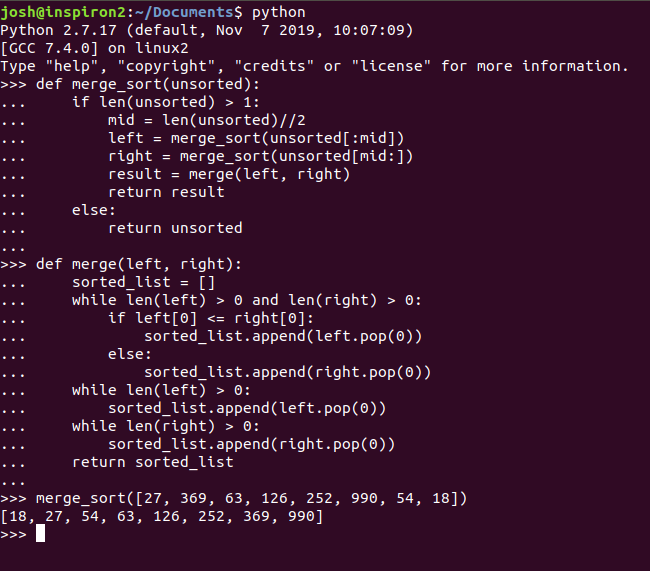然后你去！ 用Python易于阅读，简短的实现。

## 示例2：Javascript

同样，在此示例中，我们编写了第一个merge_sort()函数，以将未排序的数组分解为较小的子数组，在调用merge()函数之前，递归地调用自身，直到完成所有划分为止。

由于我们需要将其分为2个单独的数组，因此我们再次需要计算中点。 Javascript将对奇数执行浮点计算，因此我们将使用Math.floor()进行四舍五入，就像Python对//除数所做的那样。 因此，我们可以为中点设置一个变量，创建左右数组，然后在调用merge()之前将它们传递回merge_sort() merge()

const merge_sort = ( function ( unsorted ) {
var mid = Math .floor(unsorted.length/ 2 )
var left = unsorted.slice( 0 ,mid)
var right = unsorted.slice(mid,)
})

这对于包含多个元素的数组很好用，但是当您只有一个元素时，将导致无限循环和有关未定义变量的错误。 我们必须将其包装在if语句中，仅在unsorted元素有多个的情况下才执行此代码块，而在只有一个元素的情况下仅返回该元素。 我们还需要为左右数组调用我们的merge()函数，如果unsorted数组中有多个元素，则返回该函数。

const merge_sort = ( function ( unsorted ) {
if (unsorted.length > 1 ){
var mid = Math .floor(unsorted.length/ 2 )
var left = merge_sort(unsorted.slice( 0 ,mid))
var right = merge_sort(unsorted.slice(mid,))
return merge(left, right)
} else {
return unsorted
}
})

现在我们可以开始我们的merge()函数。 我们将初始化一个数组以保存排序后的结果，而left和right数组都包含元素，我们将比较两者的第一个元素。 无论哪个元素较小，都会从该数组中移出并推入结果中。 为了使代码简短而紧凑，并使用一个简单的if/else语句，我选择使用单行三元运算。 如果您不熟悉三元运算，则它们是单行if语句，其结构如下：

(condition to evaluate) ? (do this if true ) : ( do this if false )

首先写出将在if语句中保留的表达式，然后是? 。 下一个表达式是如果被评估的语句为true时该怎么办，然后是: ，然后是该操作为false时该怎么办。 我更喜欢JavaScript中的这些，而不喜欢Python中的它们，因为我发现可读性更容易。 在Python中，语法为(do this if true) if (statement to evaluate) else (do this if false) ，将要评估的语句放在中间，并在每一侧进行所需的操作。 就个人而言，我觉得它不那么容易阅读。 这是我不喜欢的Python古怪之一，非常类似于split() vs join()语法。

因此，我们的第一个while循环将包含三元函数，以在数组之间适当地移动元素，并且我们需要再添加2个while循环，以在第一个循环之后检查左右数组中是否有剩余元素，并添加它们到排序的数组。

const merge = ( function ( left, right ) {
var sorted = []
while (left.length > 0 && right.length > 0 ){
left[ 0 ] < right [ 0 ] ? sorted.push(left.shift()) : sorted.push(right.shift())
}
while (left.length> 0 ){
sorted.push(left.shift())
}
while (right.length> 0 ){
sorted.push(right.shift())
}
return sorted
})

现在，我们完整的mergesort.js文件如下所示：

const merge_sort = ( function ( unsorted ) {
if (unsorted.length > 1 ){
var mid = Math .floor(unsorted.length/ 2 )
var left = merge_sort(unsorted.slice( 0 ,mid))
var right = merge_sort(unsorted.slice(mid,))
return merge(left, right)
} else {
return unsorted
}
})

const merge = ( function ( left, right ) {
var sorted = []
while (left.length > 0 && right.length > 0 ){
left[ 0 ] < right [ 0 ] ? sorted.push(left.shift()) : sorted.push(right.shift())
}
while (left.length> 0 ){
sorted.push(left.shift())
}
while (right.length> 0 ){
sorted.push(right.shift())
}
return sorted
})

现在，我们可以在浏览器控制台中对其进行测试，以确保其正常工作：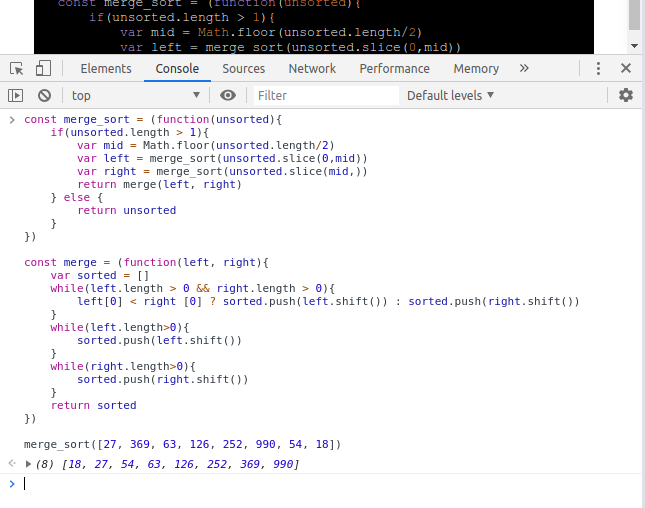## 再谈时间复杂度

现在，我们有两种使用两种不同语言实施的算法的不同示例，我们可以再次进行分析，以了解合并排序的复杂度为何以及为什么是O（n * log（n）） 。 上面的两个示例都创建了两个函数来执行算法的2个主要部分。 两种实现都使用父函数merge_sort()将其传递的列表递归地分成两半。 因此，实际的merge_sort()函数将执行几次，并且该次数随着传递给它的列表大小的对数而增加。 因此，可以说merge_sort()函数本身的复杂度为O（log（n））

另一方面，由merge_sort()调用的merge()函数的任务是获取两个输入并将它们组合为一个输出。 这将花费的时间完全取决于传递给它的输入的大小。 因此，可以说merge()函数的复杂度为O（n） 。 该merge()函数，在O（N）被调用一次 ，每次merge_sort()被调用，并且次数merge_sort()被称为是log（n）的时间，给我们提供了最后的复杂度为O（N *日志（n））

合并排序算法始终有效，这就是为什么它被用作Java和Perl等语言的默认排序算法以及Python使用的混合Timsort的一部分的原因。 时间复杂度保持恒定，这在考虑性能和优化时是一件好事，并且由于它具有将输入递归地分成两半的性质，因此如果一个人一次可以访问多个CPU，则可以高度优化该算法。

这样就可以了，合并排序。 希望在此之后，您将对该方法有扎实的了解，甚至可以在直观的水平上真正理解O（n * log（n））的实际含义。 如果您喜欢这个或学到了一些东西，请分享！

合并排序算法排序过程

展开全文• ## 分治法-合并排序

千次阅读 多人点赞 2019-08-07 20:13:02
1.合并排序 排序算法是对一组数进行顺序排序或者逆序排序，而合并排序就是排序算法的一种。合并排序用到了分治策略实现对元素进行排序。 合并排序的基本思想：把待排序的n个元素分解成n组，也就是每组一个元素；...

# 1.合并排序

排序算法是对一组数进行顺序排序或者逆序排序，而合并排序就是排序算法的一种。合并排序用到了分治策略实现对元素进行排序。

合并排序的基本思想：把待排序的n个元素分解成n组，也就是每组一个元素；之后对分好的组进行两两合并（无配对的则不操作），以此类推。

以序列{8, 3, 2, 6, 7, 1, 5, 4}为例，排序过程如下：

图片来源

合并排序又叫做2-路归并排序，是因为它每次都是两两归并。

/**
* 合并src[left:mid] src[mid+1:right] 到dest[left:right]
* @param src: 源数组
* @param dest: 目的数组
* @param left: 数组起始索引
* @param mid: 源数组的中间索引
* @param right: 目的数组的结束索引
*/
template<class Type>
void merge(Type src[], Type dest[], int left, int mid, int right) {
//指针
int i = left, j = mid + 1, k = left;
//归并
while ((i <= mid) && (j <= right)) {
if (src[i] <= src[j])
dest[k++] = src[i++];
else
dest[k++] = src[j++];
}
//把剩下的数组元素拷贝到目的数组
if (i > mid)
for (int q = j; q <= right; q++)
dest[k++] = src[q];
else
for (int q = i; q <= mid; q++)
dest[k++] = src[q];
}

merge函数用于把src[left:mid] 和src[mid + 1: right]归并到dest的对应位置。其思路如下

1. 设置两个索引变量i和j保存当前指向的src[left:mid] 和src[mid + 1: right]的索引；
2. 每次从i 和j 所指向的src[i] src[j]中取一个小值依次放到dest的对应位置，然后这个索引加一；
3. 重复2步骤直至遍历完短数组；
4. 把剩余的值拼接到dest数组中。
/**
* src[left:right]复制到dest[left:right]
* @param src: 源数组
* @param dest: 目的数组
* @param left: 起始索引
* @param right: 结束索引
*/
template<class Type>
void copy(Type src[], Type dest[], int left, int right) {
while (left < right) {
dest[left] = src[left];
left++;
}
}


copy的作用就是数组复制。

/**
* 用于合并排序好的相邻数组段
*/
template<class Type>
void mergePass(Type x[], Type y[], int step, int n) {
int i = 0;
while (i <= n - 2 * step) {
merge(x, y, i, i + step - 1, i + 2 * step - 1);
i = i + 2 * step;
}
if (i + step < n)
merge(x, y, i, i + step - 1, n - 1);
else
for (int j = i; j < n ; j++)
y[j] = x[j];
}

mergePass函数主要用于合并排序好的相邻数组，

当step为1,时，此时数组被分成了n份，mergePass的while就是把上面的单个元素两两合并；

当step为2,时，则对最多为2个元素的子数组进行两两合并。

以此类推

在之后还有一个判断：i + step < n，这里举例子来说明：

比如此时进行到了第二次归并，即step = 2, 元素如下：

x = {1,2} {2, 3}, {1, 1} {4}

i = 0时，合并的是x[0: 1]和x[2:3],之后i = 0 + 2 * 2 = 4；

i = 4,时，得到i <= 7 - 2 * 2 = 3 不成立，所以不再循环。

虽然循环不成立，但是可以看到，{1, 1} {4}还是满足i + step < n的，所以这里又加了最后一个归并。

而当x = {1, 2} {2, 3} {4}，step=2的情况下则会直接进行复制。

从上面的举例可以看出，赋予step递增的值，则可以实现归并排序。

template<class Type>
void mergeSort(Type a[], int n) {
Type* temp = new Type[n];
int step = 1;

while (step < n) {
//合并到数组temp
mergePass(a, temp, step, n);
step += step;
//合并到数组a
mergePass(temp, a, step, n);
step += step;
}
delete[] temp;
}

在mergeSort函数中，调用mergePass来实现归并操作。

接着测试一下：

int main() {
int a[] = {1, 4, 2, 6, 7, 5, 3};
int len = sizeof(a) / sizeof(a);
//合并排序
mergeSort(a, len);

for (int i = 0; i < len; i++)
cout << a[i] << " ";
cout << endl;
return 0;
}

# 2.自然合并排序

自然合并排序是上述所说的合并排序算法的一个变形。之前说过，合并排序是从一个元素开始两两归并的，而当一个组内只有一个元素时，能够保证它是排序好的。基于上面的原理，给定一个数组，一般都会存在多个长度大于1的已自然排好序的子数组段。比如对于一个数组{1, 4, 2, 6, 7, 5, 3, 2}。自然排序的子数组段为{1, 4} {2, 6, 7} {5} {3} {2}；第一次排序可以得到{1, 2, 4, 6, 7} { 3, 5} {2}；第二次排序得到{1, 2, 3, 4, 5, 6, 7} {2} ；第三次排序则可以得到递增序列。

上面需要解决的问题就是如何保存每次的子数组段的开始和结束，因为子数组段的大小不是固定的，所以还需要一个数组去维护子数组的边界。

template<class Type>
void mergeSort(Type a[], int len) {
if (len == 0)
return;
//保存子数组段的索引
vector<int> indices(len + 1);
indices = -1;
//indices中的真实个数
int number = get_order(a, len, indices);
Type* temp = new Type[len];
number = indices.size();
//自然归并排序
while (number != 2) {
int index = 0;
for (int i = 0; i + 2 < number; i += 2) {
merge(a, temp, indices[i] + 1, indices[i + 1], indices[i + 2]);
}
//回写到数组a
for (int i = 0; i < len; i++)
a[i] = temp[i];
number = get_order(a, len, indices);
}
delete[] temp;
}


新的mergeSort函数用到了子数组段，其中维护了indices数组来存储边界，

首先可以看到indices的大小为len + 1,这是最坏情况下的indices的最大值，当数组完全逆序的时候，比如要把{5, 4, 3, 2, 1}排序成{1, 2, 3, 4, 5}时，我们可以一眼看出只需要完全翻转就可以了，不过程序则不知道，按照之前的步骤来说，以上分成了{5} {4} {3} {2} {1}共5个，再加上一开始的-1，所以最大数组长度设为了len + 1。

第一个元素为-1，主要是为了应对之后的排序。以{1, 4, 2, 6, 7, 5, 3, 2}为例子。自然排序的子数组段为{1, 4} {2, 6, 7} {5} {3} {2}；那么indices的值为{-1, 1, 4, 5, 6, 7}。那么需要a[-1 + 1: 1]和a[1 + 1, 4]进行归并，a[4 +１: 5]和a[5 + 1:6]归并...

每一次的循环都会重新获取排序好的子数组段（其实可以从之前得到的）。

/**
* 获取数组a的自然排好序的子数组段，返回结束索引
* @param a: 数组
* @param len: 数组a的长度
* @param indices: 排序好的结束索引数组 需要确保长度足够
* @return: 返回indices真正的长度
*/
template<class Type>
int get_order(Type a[], int len, vector<int>& indices) {
int index = 1;
int i = 1;
while (index < len) {
//记录起点
if (a[index] < a[index - 1]) {
indices[i++] = index - 1;
}
index++;
}
indices[i++] = len - 1;
return i;
}

根据mergeSort和get_order而得到的indices数组来说，必定存在的就是-1和最后一个元素的索引，所以当indices的实际个数为2的时候，表示排序结束。

# 3.测试

本测试在leetcode上执行，题目为：排序数组

普通归并排序执行：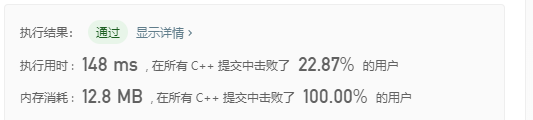自然归并排序执行：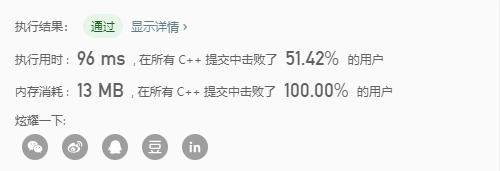可以看到，自然归并排序的执行用时要小于普通归并排序，不过内存消耗略微增加。

# 4.参考

展开全文• 主要介绍了Python选择排序、冒泡排序、合并排序代码实例,本文直接给出实现代码,需要的朋友可以参考下
• ## 合并排序（C语言）

千次阅读 2022-03-12 23:24:22
本关任务：实现合并排序算法。 编程要求 根据提示，在右侧编辑器编写代码，完成合并排序的函数。MergySort(int A[],int start, int end) ,它能将A[]数组的start到end位置的元素，用合并排序的思想完成排序。 测试...算法
• 经典排序算法的合并排序算法的C语言实现，适合初学者...